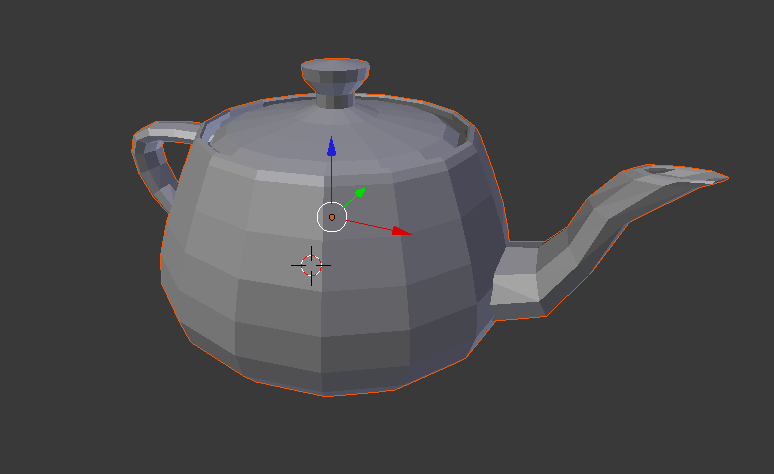# teaching machines

## I OBJect to objects

I’m going to go out on a limb here and guess that the title will have taken longer to come up with that the rest of this post…oh well.

Since we want to run some nifty graphics demos on our raspberry pi’s/ raspberries pi (no sure how to pluralize this), we thought it would be handy to have a way to loading some models. The representation we decided on was the Wavefront OBJ, as it is very popular.

Deciding that we only needed the most basic features, we limited the specs so that only triangles are accepted. Also, we only wanted to read in the  verticies and the faces, leaving the calculation of the normals up to the loader.

Here it (slightly reformatted to fit nicely):

import re

class TriMesh:

def __init__(self, fileName):
self.verts = []
self.faces = []
self.fNorms = []
self.vNorms = []

with open(fileName) as f:
for line in f:
token = re.split('\s*', line.strip())

if token == 'v':
self.verts.extend(
[float(token[i]) for i in range(1,4)])
elif line == 'f':
self.faces.extend(
[int(token[i]) - 1 for i in range(1,4)])

#initialize the vertex normals
self.vNorms =  * len(self.verts)

x,y,z = 0,1,2
for i in xrange(0, len(self.faces), 3):

#get the index of the x coordinate for the three points
A = self.faces[i] * 3
B = self.faces[i+1] * 3
C = self.faces[i+2] * 3

#create two vectors from the three points of the triangle
vBA = (self.verts[B] - self.verts[A],
self.verts[B+1] - self.verts[A+1],
self.verts[B+2] - self.verts[A+2])

vCA = (self.verts[C] - self.verts[A],
self.verts[C+1] - self.verts[A+1],
self.verts[C+2] - self.verts[A+2])

#do the cross product to get the face normal
normal = (vBA[y] * vCA[z] - vBA[z] * vCA[y],
vBA[z] * vCA[x] - vBA[x] * vCA[z],
vBA[x] * vCA[y] - vBA[y] * vCA[x])

#unitize the face normal
magnitude = (normal[x]**2  + normal[y]**2 + normal[z]**2)**.5

#dont divide by zero
if magnitude != 0:
unitNormal = (normal[x] / magnitude,
normal[y] / magnitude,
normal[z] / magnitude)
else:
unitNormal = (0.0,0.0,0.0)

#incrementally calculate vertex normals
for j in range(3):
self.vNorms[A + j] += unitNormal[j]
self.vNorms[B + j] += unitNormal[j]
self.vNorms[C + j] += unitNormal[j]

self.fNorms.extend(unitNormal)

#unitize vertex normals
for i in xrange(0, len(self.vNorms), 3):
magnitude = ( self.vNorms[i]**2 +
self.vNorms[i+1]**2 +
self.vNorms[i+2]**2)**.5

if magnitude != 0:
self.vNorms[i] /= magnitude
self.vNorms[i+1] /= magnitude
self.vNorms[i+2] /= magnitude

def printObj(self):
tmp = ''

for i in xrange(0, len(self.verts), 3):
tmp += "v  " + str(self.verts[i])
tmp +=  "  " + str(self.verts[i+1])
tmp += "  " + str(self.verts[i+2]) + "\n"
tmp += "\n"

for i in xrange(0, len(self.vNorms), 3):
tmp += "vn  "+ str(self.vNorms[i])
tmp += "  " + str(self.vNorms[i+1])
tmp += "  " + str(self.vNorms[i+2]) + "\n"
tmp += "\n"

for i in xrange(0, len(self.faces), 3):
tmp += "f  " + str(self.faces[i] + 1)
tmp += "  " + str(self.faces[i+1] + 1)
tmp += "  " + str(self.faces[i+2] + 1) + "\n"
tmp += "\n"

print tmp

The title refers to the fact that I have also wrote a version that is much more object oriented, but never quite managed to finish it.

Here’s a render, in Blender, of a teapot loaded in from a OBJ:The OBJ I used to load this,along with quite a few others, can be found at http://people.sc.fsu.edu/~jburkardt/data/obj/obj.html (the link tag keeps getting deleted for some reason)